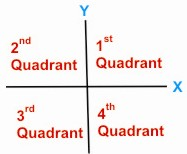HOME MATH DICTIONARY DOWNLOAD FEEDBACK DISCLAIMER
 Question: What is Quadrant ? Answer: A Circle can be cut into four equal sectors by two diameters at right angles. Each of these quarters of the circle is called a quadrant. Each of the four regions into which the x-axis and y-axis divide the plane is also called a quadrant. A quarter of a plane, bounded by two lines at right angles to one another. For example, in Cartesian co-ordinates the part of the plane with both x and y positive is a quadrant.